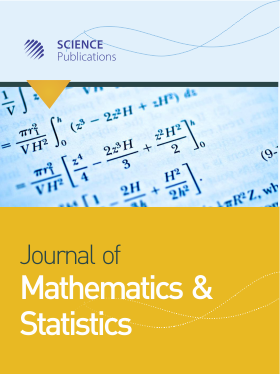Frequency: Continuous
ISSN: 1549-3644 (Print)
ISSN: 1558-6359 (Online)
Research Article Open Access

# Tilted Beta Binomial Linear Regression Model: A Bayesian Approach

Edilberto Cepeda-Cuervo1 and Maria Victoria Cifuentes-Amado2
• 1 Universidad Nacional de Colombia, Colombia
• 2 Universidad Nacional Colombia, Colombia
Journal of Mathematics and Statistics
Volume 16 No. 1, 2020, 1-8

DOI:

Submitted On: 20 November 2019
Published On: 5 January 2020

How to Cite: Cepeda-Cuervo, E. & Cifuentes-Amado, M. V. (2020). Tilted Beta Binomial Linear Regression Model: A Bayesian Approach. Journal of Mathematics and Statistics, 16(1), 1-8. https://doi.org/10.3844/jmssp.2020.1.8

## Abstract

This paper proposes new linear regression models to deal with overdispersed binomial datasets. These new models, called tilted beta binomial regression models, are defined from the tilted beta binomial distribution, proposed assuming that the parameter of the binomial distribution follows a tilted beta distribution. As a particular case of this regression models, we propose the beta rectangular binomial regression models, defined from the binomial distribution assuming that their parameters follow a beta rectangular distribution. These new linear regression models, defined assuming that the parameters of these new distributions follow regression structures, are fitted applying Bayesian methods and using the OpenBUGS software. The proposed regression models are fitted to an overdispersed binomial dataset of the number of seeds that germinate depending on the type of chosen seed and root.